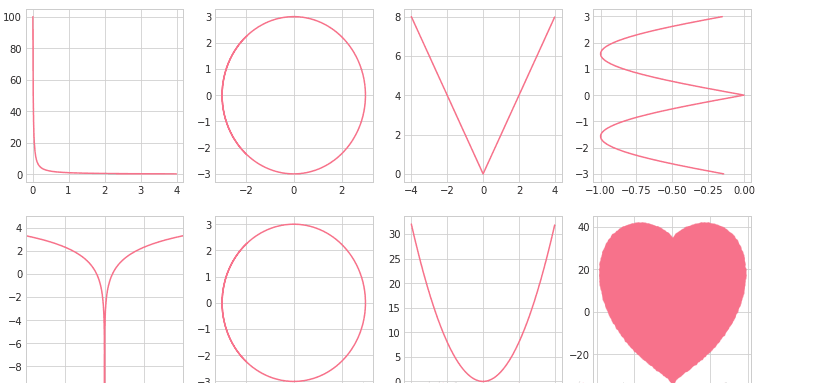• 他想知道，一个有N×N个格子的正方形棋盘，每个格子可以C种不同颜色来染色，一共可以得到多少种不同的棋盘。如果一个棋盘，经过任意旋转，反射后变成另一个棋盘，这两个棋盘就是属于同一种棋盘。 比如当N=C=2的...Golang erlang r语言
• 原标题：如何正确使用Python进行表白提到程序员，可能每个同学都认识一个——爱穿格子衬衫、冲锋衣，爱宅着敲代码的程序员或者准程序员，他们仿佛永远在bug和debug的路上，还总爱自黑自己是"单身狗”，你可能...
原标题：如何正确使用Python进行表白提到程序员，可能每个同学都认识一个——爱穿格子衬衫、冲锋衣，爱宅着敲代码的程序员或者准程序员，他们仿佛永远在bug和debug的路上，还总爱自黑自己是"单身狗”，你可能认为他们是这样的：这样的还有这样的其实不然一些程序员表面上看似孤身一人其实人家已经悄咪咪的找了女朋友单身的朋友依旧独自行走在冬夜的冷风中总不能用代码写一个女朋友出来吧程序员版本《恋爱宝典》你值得拥有表白的错误示范大胆冒失型：缺根筋型：用力过猛型：其实程序员们也有属于自己的浪漫啦，譬如Python、C、Java、C#等等等~都有很强悍的撩妹功能。本期，小编为大家带来使用Python表白的各种姿势。如果再学不会，那就真的是凭自己的本事单身了！01初阶版：一行代码画出爱心♡使用语言：Python代码：print('n'.join([''.join([('Love umd'[(x-y)%8]if((x*0.05)**2+(y*0.1)**2-1)**3-(x*0.05)**2*(y*0.1)**3<=0 else' ')for x in range(-30,30)])for y in range(15,-15,-1)])效果图：02进阶版：python + 数学= LOVE YOU使用语言：Python代码：import matplotlib.pyplot as pltimport seabornimport numpyl = numpy.arange(0, 4, 0.01)L = 1.0 / ltheta = numpy.arange(-4, 4, 0.01)o = 3.0 * numpy.cos(theta)O = 3.0 * numpy.sin(theta)v = numpy.arange(-4, 4, 0.01)V = numpy.abs(-2.0 * v)e = numpy.arange(-3, 3, 0.01)E = -1.0 * numpy.abs(numpy.sin(e))y = numpy.arange(-10, 10, 0.01)Y = numpy.log2(numpy.abs(y))u = numpy.arange(-4, 4, 0.01)U = 2.0 * u ** 2points = []for heartY in numpy.linspace(-100, 100, 500):for heartX in numpy.linspace(-100, 100, 500):if ((heartX*0.03)**2+(heartY*0.03)**2-1)**3-(heartX*0.03)**2*(heartY*0.03)**3 <= 0:points.append({"x": heartX, "y": heartY})heart_x = list(map(lambda point: point["x"], points))heart_y = list(map(lambda point: point["y"], points))fig = plt.figure(figsize=(13, 7))ax_L = fig.add_subplot(2, 4, 1)ax_O = fig.add_subplot(2, 4, 2)ax_V = fig.add_subplot(2, 4, 3)ax_E = fig.add_subplot(2, 4, 4)ax_Y = fig.add_subplot(2, 4, 5)ax_O_2 = fig.add_subplot(2, 4, 6)ax_U = fig.add_subplot(2, 4, 7)ax_heart = fig.add_subplot(2, 4, 8)plt.plot(colos='tomato')ax_L.plot(l, L)ax_O.plot(o, O)ax_V.plot(v, V)ax_E.plot(E, e)ax_Y.plot(y, Y)ax_Y.axis([-10.0, 10.0, -10.0, 5.0])ax_O_2.plot(o, O)ax_U.plot(u, U)ax_heart.scatter(heart_x, heart_y, s=10, alpha=0.5)seaborn.set_style('whitegrid')plt.show()效果图：03终极版：用Python画一只玫瑰使用语言：Python代码：import turtleimport time# writing txtturtle.hideturtle()turtle.penup()turtle.goto(130, 50)# turtle.pendown()turtle.color("blue")turtle.write("亲爱的，给你画个东西", font=("Times", 18, "bold"))time.sleep(2)turtle.goto(180, 10)turtle.write("开始了哈~", font=("Times", 18, "bold"))time.sleep(2)turtle.goto(200, -20)turtle.write("Ready?", font=("Times", 18, "bold"))time.sleep(2)turtle.goto(215, -50)turtle.write("go!", font=("Times", 18, "bold"))time.sleep(2)# turtle.end_fill()## 设置初始位置turtle.goto(0, 0)turtle.color("black")turtle.penup()turtle.left(90)turtle.fd(200)turtle.pendown()turtle.right(90)# 花蕊turtle.fillcolor("red")turtle.begin_fill()turtle.circle(10, 180)turtle.circle(25, 110)turtle.left(50)turtle.circle(60, 45)turtle.circle(20, 170)turtle.right(24)turtle.fd(30)turtle.left(10)turtle.circle(30, 110)turtle.fd(20)turtle.left(40)turtle.circle(90, 70)turtle.circle(30, 150)turtle.right(30)turtle.fd(15)turtle.circle(80, 90)turtle.left(15)turtle.fd(45)turtle.right(165)turtle.fd(20)turtle.left(155)turtle.circle(150, 80)turtle.left(50)turtle.circle(150, 90)turtle.end_fill()# 花瓣1turtle.left(150)turtle.circle(-90, 70)turtle.left(20)turtle.circle(75, 105)turtle.setheading(60)turtle.circle(80, 98)turtle.circle(-90, 40)# 花瓣2turtle.left(180)turtle.circle(90, 40)turtle.circle(-80, 98)turtle.setheading(-83)# 叶子1turtle.fd(30)turtle.left(90)turtle.fd(25)turtle.left(45)turtle.fillcolor("green")turtle.begin_fill()turtle.circle(-80, 90)turtle.right(90)turtle.circle(-80, 90)turtle.end_fill()turtle.right(135)turtle.fd(60)turtle.left(180)turtle.fd(85)turtle.left(90)turtle.fd(80)# 叶子2turtle.right(90)turtle.right(45)turtle.fillcolor("green")turtle.begin_fill()turtle.circle(80, 90)turtle.left(90)turtle.circle(80, 90)turtle.end_fill()turtle.left(135)turtle.fd(60)turtle.left(180)turtle.fd(60)turtle.right(90)turtle.circle(200, 60)time.sleep(2)turtle.penup()turtle.color("blue")turtle.goto(180, -100)turtle.write("umd~送给你~", font=("Times", 18, "bold"))time.sleep(20)效果图：怎么样？各位霸霸们，get到新技能了吗还有什么新奇的招数热烈欢迎程序猿霸霸们在留言板给大家提点一番编辑丨赵奉超图源 | 梦游家、网络责任编辑：
展开全文• 他想知道，一个有N×N个格子的正方形棋盘，每个格子可以C种不同颜色来染色，一共可以得到多少种不同的棋盘。如果一个棋盘，经过任意旋转，反射后变成另一个棋盘，这两个棋盘就是属于同一种棋盘。 比如当N=C=2的...Golang erlang r语言
• HSSFCell excel的格子单元 HSSFFont excel字体 HSSFDataFormat 日期格式 在poi1.7中才有以下2项： HSSFHeader sheet头 HSSFFooter sheet尾（只有打印的时候才能看到效果） 和这个...
• 网络工程师简介 ...今天讲讲Console线怎么用。首先Console接口是典型的配置接口，然后使用Console线直接连接至笔记本电脑的USB口或蓝牙，再利用终端仿真程序在本地配置网络设备。 Console线 网络设备的Co
网络工程师简介

网络工程师的标准打扮是穿立领的格子衫和泛白的牛仔裤，脚踏登山鞋，背着吃饭的家伙—电脑包。一整套的商务休闲风，既可以见客户做交流、讲PPT，也可以去机房扛设备、做网线。电脑包里面必备ThinkPad笔记本电脑、网线、Console线，称职的网络工程师还会带上水晶头、网线钳和测线仪。今天讲讲Console线怎么用。首先Console接口是典型的配置接口，然后使用Console线直接连接至笔记本电脑的USB口或蓝牙，再利用终端仿真程序在本地配置网络设备。Console线
网络设备的Console接口一般有两种：一种是常见的RJ-45接口，一种是Mini-usb接口。RJ-45接口可以使用三种方式连接：
1、USB转串口线 + 串口转RJ-45调试线：需要安装串口线驱动，才可以使用。2、无线蓝牙转串口线 + 串口转RJ-45调试线：笔记本连上无线蓝牙即可使用。3、USB转RJ-45调试线：需要安装官网驱动，才可以使用。Mini-usb接口可以使用Mini-usb to USB线进行连接，安装官网驱动即可使用。最后检查设备管理器中COM口是否正常。查看方法：右键“我的电脑”—点击“管理”—点击“设备管理器”—点击“端口(COM和LPT)”—查看COM口状态。配置Console口
在PC上打开终端仿真软件，常用的软件有SecureCRT和PuTTY。我们以SecureCRT为例，点击“新建连接”图标，设置连接的接口以及通信参数。单击“连接”进入命令行操作视窗，按回车键开始操作。关注公众号：网络技术平台，回复“资料”获取视频、培训教程、实验手册、电子书。展开全文网络
• 2、其实用DFS就可以做了，有人说拓扑排序，大同小异，拓扑排序也是dfs啊，而且题不可能有环，也就是不可能有互相调用的两个格子，所以放心dfs。采用记忆化搜索可以提高时间效率。 3、题还了ato
1、好题啊~涉及的知识点很多，要吐槽一下的就是题目比较吓人，列最多达到ZZZ，那数组可能有18000列！怎么想都RE吧~看了别人的题解，发现只要开到1010就可以了，这题目，纯粹是忽悠人。。。
2、其实用DFS就可以做了，有人说用拓扑排序，大同小异，拓扑排序也是dfs啊，而且本题不可能有环，也就是不可能有互相调用的两个格子，所以放心dfs。采用记忆化搜索可以提高时间效率。
3、本题还用了atoi函数，还用了string类（真方便），还用了结构体来表示每个格子，总之各种方便~
4、一个教训就是我刚开始把字母化为数字的时候，以为A是0，B是1，结果一直RE，后来我想可不是嘛，如果这样的话，那AAA还是0，就和A冲突了，这样乱七八糟地搞混，当然很容易交错访问，继而RE了。其实A是1，Z是26，那AA就是27~这点和十进制又不太一样，真的很微妙。
5、还有就是预处理，在字符串后加个“+”，以“+”为处理的标志，很巧妙。注意每次处理后，trow，tcol都要归零。
6、至此，数据结构部分结束。
#include<iostream>
#include<cstdio>
#include<cstdlib>
#include<cctype>
#include<cstring>
using namespace std;
typedef struct{
bool type;
string s;
int num;
}table;
table a;
int dfs(int i,int j){
if(a[i][j].type) return a[i][j].num;
int trow=0,tcol=0,p=0;
for(int p=0;p<a[i][j].s.size();p++){
if(isdigit(a[i][j].s[p])) trow=trow*10+a[i][j].s[p]-'0';
else if(isalpha(a[i][j].s[p])) tcol=tcol*26+a[i][j].s[p]-'A'+1;
else if(a[i][j].s[p]=='+'){a[i][j].num+=dfs(trow-1,tcol-1);trow=0;tcol=0;}
}
a[i][j].type=true;
return a[i][j].num;
}
int main(){
int T,col,row;
scanf("%d",&T);
while(T--){
scanf("%d%d",&col,&row);
for(int i=0;i<row;i++)
for(int j=0;j<col;j++){
cin>>a[i][j].s;
if(a[i][j].s=='='){
a[i][j].type=false;
a[i][j].num=0;
a[i][j].s+="+";
}
else{
a[i][j].type=true;
a[i][j].num=atoi(a[i][j].s.c_str());
}
}
for(int i=0;i<row;i++){
for(int j=0;j<col;j++){
printf("%d",dfs(i,j));
if(j!=col-1) printf(" ");
}
printf("\n");
}
}
return 0;
}

展开全文• 题意：给你一个n*n的格子，然后是k个坐标，每个坐标代表这个地方有一个小行星。每个光束可以摧毁一整行或一整列的小行星，求...最大匹配和最小顶点覆盖就是两个概念，并没有什么关系，如果一开始想怎么匹配，那完

http://poj.org/problem?id=3041
题意：给你一个n*n的格子，然后是k个坐标，每个坐标代表这个地方有一个小行星。每个光束可以摧毁一整行或一整列的小行星，求问最少用多少光束可以摧毁所有小行星。

思路：好吧。。刚开始愣是不知道怎么建图，这种问题居然能和最小顶点覆盖联系到，学到了。最大匹配和最小顶点覆盖本就是两个概念，并没有什么关系，如果一开始想怎么匹配，那完蛋了。直接找怎样用最少顶点覆盖全图才是王道。
把每行看成一个顶点，每列看成一个顶点，中间的小行星就代表该行和该列有联系，也就是两个顶点有边。类似于邻接矩阵又不全是。那么一行上有多少行星就代表以这个节点为起点有哪些边，于是可以被一束光束清除，这一束光束即覆盖这些边，当做覆盖定点，求最小值和找增广路的操作类似，直接用匹配的算法即可。说真的写到现在我觉得最小顶点覆盖也就和最大匹配数值相等而已= =。

#include <stdio.h>
#include <algorithm>
#include <stdlib.h>
#include <string.h>
#include <iostream>
#include <queue>
#include <stack>
#include <ctype.h>

using namespace std;

typedef long long LL;

const int N = 505;
const int INF = 0x3f3f3f3f;

int head[N], match[N];
bool vis[N];
int n, m, cnt;

struct Edge
{
int to,next;
}edge[N*N];

void add(int u, int v)
{
edge[cnt] = (struct Edge){v, head[u]};
head[u] = cnt++;
}

void init()
{
cnt = 0;
memset(head, -1, sizeof(head));
}

bool Augment(int u)
{
for(int i = head[u]; i != -1; i = edge[i].next)
{
int v = edge[i].to;
if(!vis[v])
{
vis[v] = true;
if(match[v]==-1 || Augment(match[v]))
{
match[v] = u;
return true;
}
}
}
return false;
}

int Hungary(int n)
{
int ans = 0;
memset(match, -1, sizeof(match));
for(int i = 1; i <= n; i++)
{
memset(vis, false, sizeof(vis));
if(Augment(i)) ans++;
}
return ans;
}

int main()
{
//  freopen("in.txt", "r", stdin);
int n, u, v, k;
scanf("%d%d", &n, &k);
init();
for(int i = 1; i <= k; i++)
{
scanf("%d%d", &u, &v);
add(u, v);
}
printf("%d\n", Hungary(n));
return 0;
}

展开全文• 重庆大学城北大青鸟带大家一起来看看，程序员的十二时辰，是怎么捉狼的！ 一、巳初 带上武器（双肩背包），穿好战袍（格子衫），早上9点，准时到达公司；既不太早也不太迟，这是顶级程序员精准的时间意识。 浸泡一杯...
• 回到宿舍自己拿PS弄（软件电脑有，一直闲置，终于能上了），网上找了一圈，发现没有一个完整的教程，要么软件版本不对导致的命令位置不同（分cc版和cs版本），要不就是改完白边严重，不知道怎么修改。 东拼西凑，...
• 篇总结不具体讲解A*算法的实现以及原理，主要告诉怎么用。后面的总结中我会陆续把A*算法进行详细分析，希望大家学习。2. 开发流程2.1. 地图加载1)在寻路过程中，地图是被分为若干个小格子，当然，根据格子数量的...flex flash 算法 timer 任务
• 题意是给你一个由 p，q 组成一个矩形的棋盘，让你马棋在这上面走，是否能一线走完这 p * q 个格子。 关于这条路线是怎么走的，自己动手在纸上模拟一下样例3棋子行走的过程就可以了。 所以这种一线走完的题意可以很...
• 生成完后，再一个循环去扫描这些-1的格子，把它周边的格子都加1，当然必须是非金子的格子才加1。代码放在这里。 其中increaseArround用来把这格金子周边的格子都加1，实现也比较简单： 执行genMimeArr()，随机...
• 补充：由于边界被窗口上面那一行挡住了，所以我给程序动了一个小手术，加上了一个panel，但是随之带来了一个问题，pack（）设置大小的话窗口只是一条线，没有办法让窗口刚刚好将Panel包住，如果有大牛知道怎么弄...java 贪吃蛇
• 好了，让我们看看它是怎么运作的。我们最初的9格方格中，在起点被切换到关闭列表中后，还剩8格留在开启列表中。这里面，F值最低的那个是起始格右侧紧邻的格子，它的F值是40。因此我们选择这一格作为下一个要处理的...算法
• 游戏中的各种形状及整个游戏空间怎么用数据表示？ 游戏中怎么判断左右及向下移动的可能性？ 游戏中怎么判断某一形状旋转的可能性？ 按向下方向键时加速某一形状下落速度的处理？ 怎么判断某一形状已经到底？ ...C语言 俄罗斯方块
• 怎么确定粒子的摆放位置？ <p>emmmm作者目前想到的办法是：降低像素数</strong></p> 来看下这个“非常粗略”的示意图： <p><img alt="image" src=...
• 关於通讯的指令.........................................................................................................21 十. 编译器( Compiler ).......................................................linux
• 系统是在Windows XP系统和MapGis6.7(B20051118)基础上，以Microsoft VC++ 6.0为编程语言，MapGis 6.7 SDK为开发平台进行开发的地质图件制作软件。系统基于MapGis输入编辑子系统强大的图形编辑能力，添加专业的地质...MAPGIS